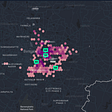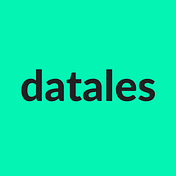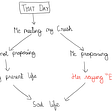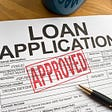# Predicting house prices with linear regression

Inspired by Kevin Markham’s post on linear regression, Chapter 3 of An Introduction to Statistical Learning and Andrew Ng’s Machine Learning course.

We’ll be working with a dataset of house prices from Kaggle.

## Simple Linear Regression

Simple linear regression is a statistical approach for modelling the relationship between a predictor variable X and a response variable Y. It assumes there is a linear relationship between these two variables and we use that to predict a quantitative output.

Simple linear regression is a very simple approach for supervised learning. But, even though it’s probably the simplest and most straightforward method, it’s the fundamental starting point for all regression methods, so it’s important to fully understand what it is about. It’s also widely used and quite easy to interpret: useful to get a better understanding of the relationship between the response and predictor.

Mathematically, we can write this linear relationship as:

y = β0 + β1x+ e; where

• y is the output variable (also called response, target or dependent variable). e.g. house prices
• x is the input variable (also called feature, explanatory or independent variable) e.g. size of a house in squared meters
• β0 is the intercept (the value of y when x=0)
• β1 is the coefficient for x and the slope of the regression line (“the average increase in Y associated with a one-unit increase in X”)
• e is the error term

When implementing linear regression, the algorithm finds the line of best fit using the model coefficients β0 and β1, such that it is as close as possible to the actual data points (minimises the sum of the squared distances between each data point and the line). Once we find β0 and β1 we can use the model to predict the response.

• Black dots are the observed values of x and y (actual data)
• the blue line — line of best fit — is the line that minimises the sum of squared errors
• the red lines are the errors (or residuals) — the vertical distances between the observed values (the actual data) and the line of best fit
• the slope of the blue line is β1
• β0 is the intercept (the value of y when x=0)

## An Example: Predicting house prices with linear regression using scikit-learn

Setting the environment:

`import pandas as pdimport numpy as npimport seaborn as snsimport matplotlib.pyplot as plt%matplotlib inlinefrom sklearn.linear_model import LinearRegressionfrom sklearn.model_selection import train_test_split, cross_val_scorefrom sklearn.metrics import mean_squared_error`

`houses = pd.read_csv("kc_house_data.csv")houses.head()`

Data dictionary

0. Data cleansing and exploratory analysis

`#check for nulls in the datahouses.isnull().sum() `
`# check for any correlations between variablescorr = houses.corr()sns.heatmap(corr)# sqft_living, grade, sqft_above and sqft_living15 seem to have a # high influence in price`

Building a linear regression model

1. Prepare the data: define predictor and response variables
`# create x and yfeature_cols = 'sqft_living' x = houses[feature_cols] # predictory = houses.price # response`

2. Split data into train and test

The train/test split consists in randomly making 2 subsets of the the data: the training set, used to fit our learning algorithm so it learns how to predict, and the test set which we use to get an idea of how the model would perform with new data.

`# split data into train and testx_train, x_test, y_train, y_test = train_test_split(    x, y, test_size=0.2) # the test set will be 20% of the whole data set`

3. Fit the model on the training set

`# instantiate, fitlinreg = LinearRegression()linreg.fit(x_train, y_train)`

4. Print the coefficients

`print linreg.intercept_print linreg.coef_-46773.6549892[282.29917574] # for an increase of 1 square meter in house size,# the house price will go up by ~\$282, on average`

The intercept (β0) is the value of y when x=0. In this case it would be the price of a house when the sqft_living is 0. (Note it does not always make sense to interpret the intercept). The coefficient of β1 is the change in y divided by change in x (i.e the derivative, the slope of the line of best fit). An increase of 1 square meter in house size is associated with a price increase of \$282.3, on average. Note that association doesn’t always imply causation.

5. Predict the price of a 1000 sqft_living house using our model:

`# manuallyprice = -46773.6549892 + 1000*282.29917574# using the modellinreg.predict(1000)array([ 238175.93397914])`

6. Compute the Root Mean Squared Error (RMSE), which is a commonly used metric to evaluate regression models, on the test set:

`mse = mean_squared_error(y_test, linreg.predict(x_test))np.sqrt(mse)259163.48398162922 # not greatlinreg.score(x_test,y_test)0.55433142764860421`

We get a root mean squared error of \$259,163.48 when predicting a price for a house, which is really high. This is kind of expected since we’re only using one feature in our model, and it could be greatly improved by adding more features such as number of bathrooms or bedrooms. We can also see that we’re omitting relevant variables by looking at the R squared coefficient: 55%. This means that our model is only able to explain 55% of the variability in house prices.

When we use multiple predictors (or features) we call it Multiple linear regression and we’ll be looking into it in another post.

--

--

--

Tales about data, statistics, machine learning, visualisation, and much more. By Adrià Luz (@adrialuz) and Sara Gaspar (@sargaspar).

Love podcasts or audiobooks? Learn on the go with our new app.

## Ridge Regression — A graphical tale of two concepts## Why are Geospatial Visualizations & Heatmaps Critical for Food Tech Companies?## Learn R With Baseball Data: Top First Basemen Hitters of 2021 (In Hot Weather)## Six Things You Need to Know About Changing from Business Person to Data Scientist## footiehound## Datanieuws binnen en buiten het Rijk 01–05–2018## Analyzing the World of Whisky## The Subtle Art of Successful Quantitative TradingTales about data, statistics, machine learning, visualisation, and much more. By Adrià Luz (@adrialuz) and Sara Gaspar (@sargaspar).

## DIY Gradient Descending Linear Regression## The Ultimate Guide for Evaluating Classification Models## Decision Trees, Bootstrap Aggregating and Bagging.## Predicting Personal Loan defaulter